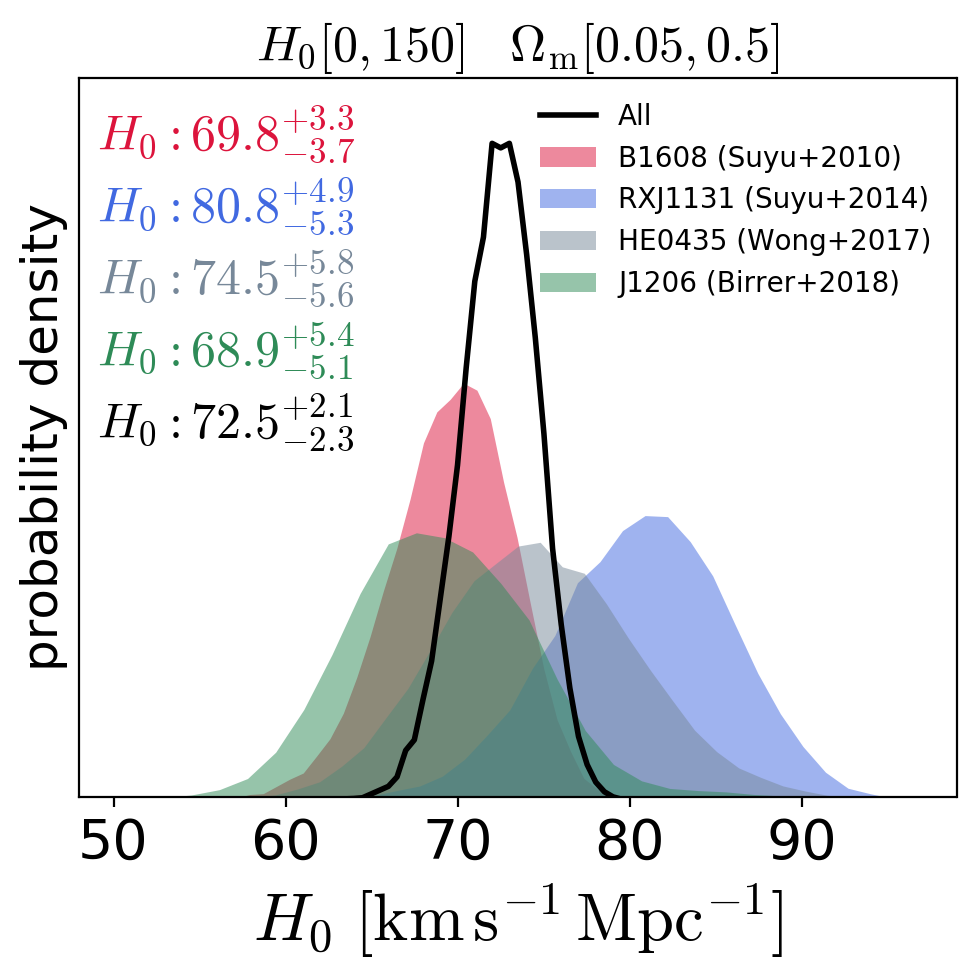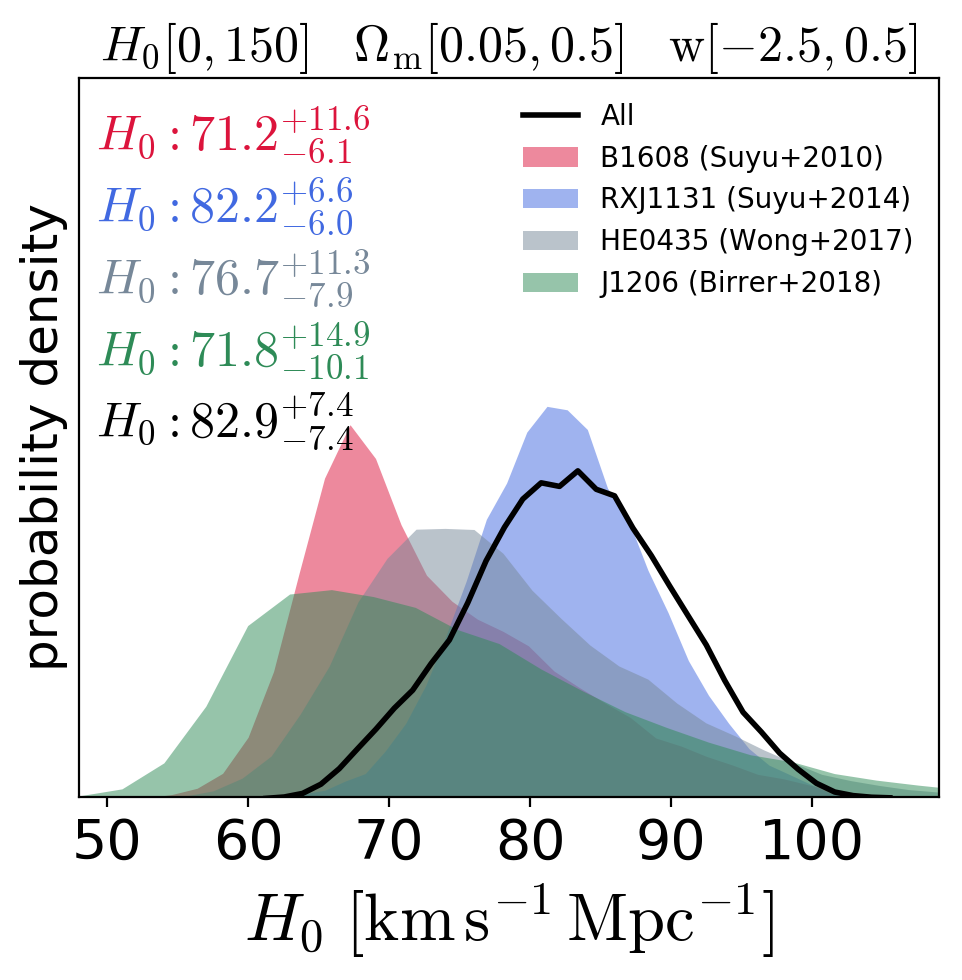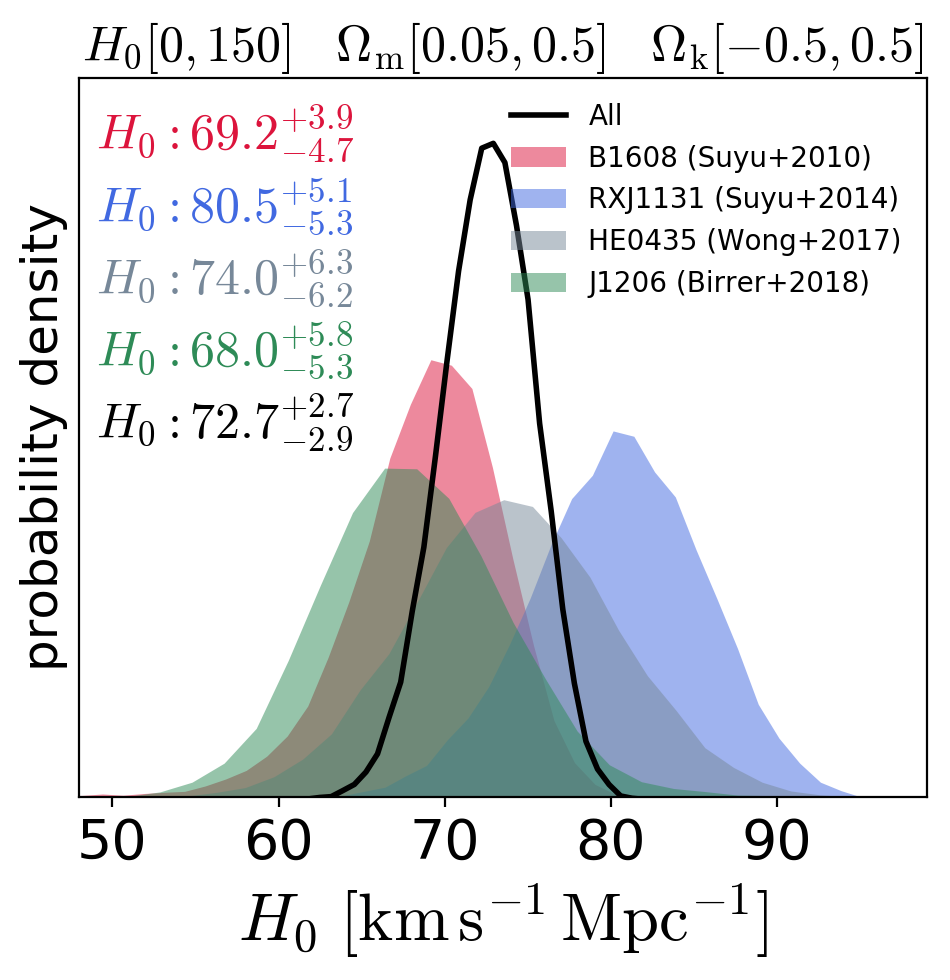# Compute H0 from strong lenses only

Vivien Bonvin, Simon Birrer, 01.2019

This notebook combines GLEE and Lenstronomy lens models output results. It samples the cosmological parameters in a chosen cosmology and makes a nice H0 inference plot.

The cores functions used here are defined in lensutils.

In :
import numpy as np
import pandas as pd
import os
import matplotlib.pyplot as plt
import lensutils as lu


We start by creating "Lens output" objects for each of the strong lens in our analysis. They are labelled GLEE lenses or Lenstronomy lenses, following the kind of output provided by the modeling code we used. More details are given in lensutils.

The relevant parameter of the various lens systems can be found here:

The J1206 angular diameter distance posteriors that we use below can be found here. If you wish to access the modeling and analysis scripts for the J2016 analysis, please get in touch with Simon Birrer.

In :
"""Create the lenses objects"""

HE0435 = lu.GLEELens(name="HE0435", longname="HE0435 (Wong+2017)", zlens=0.4546, zsource=1.693,
mu=7.57930024e+00, sigma=1.03124167e-01, lam=6.53901645e+02,
loglikelihood_type="sklogn_analytical"
)

RXJ1131 = lu.GLEELens(name="RXJ1131", longname="RXJ1131 (Suyu+2014)", zlens=0.295, zsource=0.654,
mu=6.4682, sigma=0.20560, lam=1388.8,
loglikelihood_type="sklogn_analytical"
)

B1608 = lu.GLEELens(name="B1608", longname="B1608 (Suyu+2010)", zlens=0.6304, zsource=1.394,
mu=7.0531390, sigma=0.2282395, lam=4000.0,
loglikelihood_type="sklogn_analytical"
)

J1206 = lu.LenstronomyLens(name="J1206", longname="J1206 (Birrer+2018)", zlens=0.745, zsource=1.789,
ddt_vs_dd_samples=ddt_vs_dd_1206s,
loglikelihood_type="kde", kde_type="scipy_gaussian",
)

lenses = [B1608, RXJ1131, HE0435, J1206]


The cell below contains a couple of useful flag. Leave them all True by default - it will save the MCMC chains in a samples/chosen-cosmology/nwalkers-x-nsamples folder, for later use.

In :
# True means I also sample the cosmological posteriors for each lens individually
compute_individual_samples = True

# True means the MCMC chains computed with emcee are saved on the disk
saveresults = True

# Where do you want to save your chains
samplesdir = "samples"

# Small numbers just for testing purposes. Should be increased if you make to have robust estimates.
nwalkers = 32  #32
nsamples = 20000  #20000


The actual MCMC sampling is done in the cell below. This can take quite some time depending on nsamples and nwalkers so you might want to adjust them.

Small chains (6 walkers, 7k samples each) are produced quickly, but are somewhat poorly sampled. You might want to increase the number of walkers to 32 and number of samples to 20000, for example, if you want to produce smoother plots.

In :
for cosmology in ["FLCDM", "FwCDM", "oLCDM"]:
display("Sampling cosmological parameters in %s..." % cosmology)

savedir = os.path.join(samplesdir, cosmology, "%ix%i" % (nwalkers, nsamples))

if not os.path.exists(savedir):
display("Creating directory %s" % savedir)
os.makedirs(savedir)

combined_samples = lu.sample_params(lenses, cosmology=cosmology, nwalkers=nwalkers,
nsamples=nsamples, save=saveresults,
filepath=os.path.join(savedir, "%s_samples.pkl"
% ("+".join([l.name for l in lenses]))))

if compute_individual_samples:
for lens in lenses:
lu.sample_params([lens], cosmology=cosmology, nwalkers=nwalkers,
nsamples=nsamples, save=saveresults,
filepath=os.path.join(savedir, "%s_samples.pkl" % lens.name))

'Sampling cosmological parameters in FLCDM...'
100%|██████████| 20000/20000 [35:21<00:00, 10.08it/s]
0%|          | 4/20000 [00:00<08:40, 38.39it/s]
('Autocorrelation time: ', array([ 33.49386229,  39.48529052]))

100%|██████████| 20000/20000 [29:49<00:00, 11.18it/s]
0%|          | 5/20000 [00:00<07:57, 41.88it/s]
('Autocorrelation time: ', array([ 34.16792194,  39.73130368]))

100%|██████████| 20000/20000 [07:12<00:00, 46.22it/s]
0%|          | 3/20000 [00:00<11:19, 29.43it/s]
('Autocorrelation time: ', array([ 34.98400664,  40.32251507]))

100%|██████████| 20000/20000 [06:54<00:00, 48.26it/s]
0%|          | 0/20000 [00:00<?, ?it/s]
('Autocorrelation time: ', array([ 34.77292955,  40.21808864]))

100%|██████████| 20000/20000 [23:48<00:00, 13.45it/s]

('Autocorrelation time: ', array([ 33.6871659 ,  40.76296179]))

'Sampling cosmological parameters in FwCDM...'
100%|██████████| 20000/20000 [39:34<00:00,  8.42it/s]
0%|          | 4/20000 [00:00<08:30, 39.17it/s]
('Autocorrelation time: ', array([ 57.50806247,  55.31146637,  66.56939276]))

100%|██████████| 20000/20000 [06:35<00:00, 52.99it/s]
0%|          | 4/20000 [00:00<09:19, 35.73it/s]
('Autocorrelation time: ', array([ 98.81875065,  76.07535304,  98.54341512]))

100%|██████████| 20000/20000 [06:55<00:00, 48.19it/s]
0%|          | 4/20000 [00:00<08:31, 39.08it/s]
('Autocorrelation time: ', array([ 55.06861857,  66.25218706,  60.99470923]))

100%|██████████| 20000/20000 [06:09<00:00, 54.12it/s]
0%|          | 0/20000 [00:00<?, ?it/s]
('Autocorrelation time: ', array([ 66.1399994 ,  65.7526418 ,  72.59996415]))

100%|██████████| 20000/20000 [21:49<00:00, 15.28it/s]

('Autocorrelation time: ', array([ 71.58803245,  62.26707616,  65.2921431 ]))

'Sampling cosmological parameters in oLCDM...'
100%|██████████| 20000/20000 [34:52<00:00,  8.67it/s]
0%|          | 3/20000 [00:00<11:57, 27.87it/s]
('Autocorrelation time: ', array([ 51.05690249,  61.14700533,  60.41580565]))

100%|██████████| 20000/20000 [08:02<00:00, 36.58it/s]
0%|          | 4/20000 [00:00<10:16, 32.43it/s]
('Autocorrelation time: ', array([ 94.94748898,  72.01606067,  69.51879489]))

100%|██████████| 20000/20000 [07:36<00:00, 43.77it/s]
0%|          | 4/20000 [00:00<10:14, 32.56it/s]
('Autocorrelation time: ', array([ 52.33784215,  60.84504246,  59.39425462]))

100%|██████████| 20000/20000 [07:21<00:00, 46.84it/s]
0%|          | 0/20000 [00:00<?, ?it/s]
('Autocorrelation time: ', array([ 50.81375318,  59.01754858,  64.7470877 ]))

100%|██████████| 20000/20000 [22:34<00:00, 14.76it/s]

('Autocorrelation time: ', array([ 51.20278325,  59.37543141,  60.77690787]))


The cell below will read the saved samples produced by the MCMC sampling above, and make fancy H0 probability plots out of them.

It reads by default the samples generated with the nwalkers and nsamples parameters defined two cells above.

In :
for cosmology in ["FLCDM", "FwCDM", "oLCDM"]:

savedir = os.path.join(samplesdir, cosmology, "%ix%i" % (nwalkers, nsamples))
samples_list = [lu.readpickle(os.path.join(savedir, "%s_samples.pkl" % lens.name)) for lens in lenses]
% ("+".join([l.name for l in lenses])))))

### plot nice H0s histogram
plt.figure(figsize=(5, 5), dpi=200)
plt.subplot(1, 1, 1)

H0s_list = [[s for s in samples] for samples in samples_list]
oms_list = [[s for s in samples] for samples in samples_list]

percentiles = [16, 50, 84]
colors = ["crimson", "royalblue", "lightslategrey", "seagreen", "black"]
nbins = 35
title = r"${{{0}}}_{{-{1}}}^{{+{2}}}$"
fmt = "{{0:{0}}}".format(".1f").format

# loop over the lenses, shaded histograms
for il, lens in enumerate(lenses):

h, be = np.histogram(H0s_list[il], bins=nbins, density=True)
xs = [(b+be[ind+1])/2. for ind, b in enumerate(be[:-1])]
plt.plot(xs, h, alpha=0.5, color=colors[il], linewidth=0.0)
plt.fill_between(xs, h, alpha=0.5, color=colors[il], linewidth=0.0, label=r'%s' % lens.longname)

pcs = np.percentile(H0s_list[il], q=percentiles)
txt = r'$H_{0}:$' + title.format(fmt(pcs), fmt(pcs-pcs), fmt(pcs-pcs))
plt.annotate(txt, xy=(0.0, 0.0), xytext=(0.02, 0.9-0.1*il), xycoords='axes fraction',
fontsize=18, color=colors[il])

# plot the combined result
h, be = np.histogram(H0s_list[-1], bins=nbins, density=True)
xs = [(b+be[ind+1])/2. for ind, b in enumerate(be[:-1])]
plt.plot(xs, h, alpha=1.0, color=colors[-1], linewidth=2.0, label=r'All')

pcs = np.percentile(H0s_list[-1], q=percentiles)
txt = r'$H_{0}:$' + title.format(fmt(pcs), fmt(pcs - pcs), fmt(pcs - pcs))
plt.annotate(txt, xy=(0.0, 0.0), xytext=(0.02, 0.9 - 0.1 * len(lenses)), xycoords='axes fraction',
fontsize=18, color=colors[-1])

# fine tune
if cosmology == "FLCDM":
title = r"$H_{\rm{0}} \rm{[0, 150]} \ \ \ \Omega _{\rm{m}} \rm{[0.05, 0.5]}$"
elif cosmology == "FwCDM":
title = r"$H_{\rm{0}} \rm{[0, 150]} \ \ \ \Omega _{\rm{m}} \rm{[0.05, 0.5]} \ \ \ w \rm{[-2.5, 0.5]}$"
elif cosmology == "oLCDM":
title = r"$H_{\rm{0}} \rm{[0, 150]} \ \ \ \Omega _{\rm{m}} \rm{[0.05, 0.5]} \ \ \ \Omega _{\rm{k}} \rm{[-0.5, 0.5]}$"

plt.title(title, fontsize=18)
plt.xlabel(r"$H_{\rm{0}}\rm{\ [km\,s^{-1}\,Mpc^{-1}]}$", fontsize=24)
plt.ylabel("probability density", fontsize=18)
plt.yticks(fontsize=14)
plt.xticks(fontsize=20)
plt.yticks([])

legend = plt.legend()
legend.get_frame().set_alpha(0.0)
if cosmology in ["FLCDM", "oLCDM"]:
plt.xlim([48, 99])
plt.ylim([max(h)*0.005, max(h)*1.1])
elif cosmology == "FwCDM":
plt.xlim([48, 109])
plt.ylim([max(h)*0.005, max(h)*2.2])
plt.tight_layout()

plt.savefig(os.path.join(savedir, "H0.png"))
plt.show()